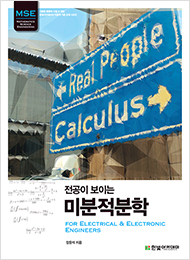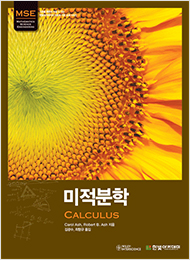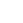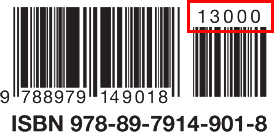# 한빛아카데미### Essential Calculus

2nd Edition | International Edition

한빛아카데미

원서

판매중

• 저자 : James Stewart
• 출간 : 2012-07-08
• 페이지 : 960 쪽
• ISBN : 9781133492566
• 물류코드 :7999
본 도서는 대학 강의용 교재로 개발되었으므로 연습문제 해답은 제공하지 않습니다.
초급 초중급 중급 중고급 고급
0점 (0명)
좋아요 : 32

Success in your calculus course starts here!

James Stewart's CALCULUS texts are world-wide best-sellers for a reason: they are clear, accurate, and filled with relevant, real-world examples.

This book is for instructors who think that most calculus textbooks are too long. In writing the book, James Stewart asked himself: What is essential for a calculus course for scientists and engineers? ESSENTIAL CALCULUS, 2E, International Metric Edition offers a concise approach to teaching calculus that focuses on major concepts, and supports those concepts with precise definitions, patient explanations, and carefully graded problems. The book is only 900 pages—two-thirds the size of Stewart's other calculus texts, and yet it contains almost all of the same topics. The author achieved this relative brevity primarily by condensing the exposition and by putting some of the features on the book's website, www.StewartCalculus.com. Despite the more compact size, the book has a modern flavor, covering technology and incorporating material to promote conceptual understanding, though not as prominently as in Stewart's other books. ESSENTIAL CALCULUS, 2E, International Metric Edition features the same attention to detail, eye for innovation, and meticulous accuracy that have made Stewart's textbooks the best-selling calculus texts in the world.저자

James Stewart

The late James Stewart received his M.S. from Stanford University and his Ph.D. from the University of Toronto. He did research at the University of London and was influenced by the famous mathematician George Polya at Stanford University. Stewart was most recently Professor of Mathematics at McMaster University, and his research field was harmonic analysis. Stewart was the author of a best-selling calculus textbook series published by Cengage Learning, including CALCULUS, CALCULUS: EARLY TRANSCENDENTALS, and CALCULUS: CONCEPTS AND CONTEXTS, as well as a series of precalculus texts.

Preface

To the Student

Diagnostic Tests

CHAPTER 1. FUNCTIONS AND LIMITS

CHAPTER 2. DERIVATIVES

CHAPTER 3. APPLICATIONS OF DIFFERENTIATION

CHAPTER 4. INTEGRALS

CHAPTER 5. INVERSE FUNCTIONS

CHAPTER 6. TECHNIQUES OF INTEGRATION

CHAPTER 7. APPLICATIONS OF INTEGRATION

CHAPTER 8. SERIES

CHAPTER 9. PARAMETRIC EQUATIONS AND POLAR COORDINATES

CHAPTER 10. VECTORS AND THE GEOMETRY OF SPACE

CHAPTER 11. PARTIAL DERIVATIVES

CHAPTER 12. MULTIPLE INTEGRALS

CHAPTER 13. VECTOR CALCULUS

APPENDIXES

INDEX

[도서 장점(마케팅 포인트)]

• The text presents exponential, logarithmic, and inverse trigonometric functions late, with the logarithm defined as an integral. Instructors wishing to cover such functions earlier should consider ESSENTIAL CALCULUS: EARLY TRANSCENDENTALS, 2e, International Metric Edition.
• Each chapter ends with a comprehensive review that includes a true/false quiz, Concept Check Questions, and exercises for every topic covered in the chapter.
• Tools for Enriching Calculus—a free, online, interactive resource that allows Calculus students to work with animations that deepen their understanding by helping them visualize the concepts they are learning—has been updated with new problems and a new Flash design that is more visually appealing and engaging. Written and narrated by James Stewart, these assignable animations are also integrated into Cengage YouBook, and many are available directly to students at www.stewartcalculus.com.
• For the convenience of instructors who wish to cover additional material, the www.stewartcalculus.com website contains: Review of Algebra, Trigonometry, Analytic Geometry, and Conic Sections; Additional Examples; Projects; Archived Problems (drill exercises from Stewart's other books) with solutions; Challenge Problems; Lies My Calculator and Computer Told Me, and more.
• Additional topics (with exercises) available at www.stewartcalculus.com include Principles of Problem Solving, Strategy for Integration, Strategy for Testing Series, Fourier Series, Area of a Surface of Revolution, Linear Differential Equations, Second Order Linear Differential Equations, Nonhomogeneous Linear Equations, Applications of Second Order Differential Equations, Using Series to Solve Differential Equations, Complex Numbers, and Rotation of Axes. Links to outside web resources and the History of Mathematics, with links to historical websites, are also available at the site.
• The text presents a concise approach to calculus for instructors who want to focus on essential principles and who feel no need for frills.
• Brevity is achieved through condensed exposition, fewer examples in some sections, fewer technology and conceptual problems, and fewer appendixes. Problems Plus and Projects have been moved to the author's website at www.stewartcalculus.com.
• Certain topics, for example, the treatment of the integral and the remainder term in Taylor Series, are presented in a manner that is more traditional than in Stewart's other books.

[부/장별 내용 요약]

Diagnostic Tests

The book begins with four diagnostic tests, in Basic Algebra, Analytic Geometry, Functions, and Trigonometry.

CHAPTER 1. FUNCTIONS AND LIMITS

After a brief review of the basic functions, limits and continuity are introduced, including limits of trigonometric functions, limits involving infinity, and precise definitions.

CHAPTER 2. DERIVATIVES

The material on derivatives is covered in two sections in order to give students time to get used to the idea of a derivative as a function. The formulas for the derivatives of the sine and cosine functions are derived in the section on basic differentiation formulas. Exercises explore the meanings of derivatives in various contexts.

CHAPTER 3. APPLICATIONS OF DIFFERENTIATION

The basic facts concerning extreme values and shapes of curves are deduced from the Mean Value Theorem. The section on curve sketching includes a brief treatment of graphing with technology. The section on optimization problems contains a brief discussion of applications to business and economics.

CHAPTER 4. INTEGRALS

The area problem and the distance problem serve to motivate the definite integral, with sigma notation introduced as needed. (Full coverage of sigma notation is provided in Appendix B.) A quite general definition of the definite integral (with unequal subintervals) is given initially before regular partitions are employed. Emphasis is placed on explaining the meanings of integrals in various contexts and on estimating their values from graphs and tables.

CHAPTER 5. INVERSE FUNCTIONS

The logarithm is defined as an integral and exponential function as its inverse. Applications to exponential growth and decay follow. Inverse trigonometric functions and hyperbolic functions are also covered here. L’Hospital’s Rule is included in this chapter because limits of transcendental functions so often require it.

CHAPTER 6. TECHNIQUES OF INTEGRATION

All the standard methods are covered, as well as computer algebra systems, numerical methods, and improper integrals.

CHAPTER 7. APPLICATIONS OF INTEGRATION

General methods are emphasized. The goal is for students to be able to divide a quantity into small pieces, estimate with Riemann sums, and recognize the limit as an integral. The chapter concludes with an introduction to differential equations, including separable equations and direction fields.

CHAPTER 8. SERIES

The convergence tests have intuitive justifications as well as formal proofs. The emphasis is on Taylor series and polynomials and their applications to physics. Error estimates include those based on Taylor’s Formula (with Lagrange’s form of the remainder term) and those from graphing devices.

CHAPTER 9. PARAMETRIC EQUATIONS AND POLAR COORDINATES

This chapter introduces parametric and polar curves and applies the methods of calculus to them. A brief treatment of conic sections in polar coordinates prepares the way for Kepler’s Laws in Chapter 10.

CHAPTER 10. VECTORS AND THE GEOMETRY OF SPACE

In addition to the material on vectors, dot and cross products, lines, planes, and surfaces, this chapter covers vector valued functions, length and curvature of space curves, and velocity and acceleration along space curves, culminating in Kepler’s laws.

CHAPTER 11. PARTIAL DERIVATIVES

In view of the fact that many students have difficulty forming mental pictures of the concepts of this chapter, I’ve placed a special emphasis on graphics to elucidate such ideas as graphs, contour maps, directional derivatives, gradients, and Lagrange multipliers.

CHAPTER 12. MULTIPLE INTEGRALS

Cylindrical and spherical coordinates are introduced in the context of evaluating triple integrals.

CHAPTER 13. VECTOR CALCULUS

The similarities among the Fundamental Theorem for line integrals, Green’s Theorem, Stokes’ Theorem, and the Divergence Theorem are emphasized.

• 첫번째 리뷰어가 되어주세요.
••••미적분학

Carol Ash , Robert B. Ash

결재하기
• 문화비 소득공제 가능

배송료 안내

• 책, 아이템 등 상품을 1만원 이상 구매시 무료배송
• 브론즈, 실버, 골드회원이 주문하신 경우 무료배송

무료배송 상품을 포함하여 주문하신 경우에는 구매금액에 관계없이 무료로 배송해 드립니다.리뷰쓰기

* 도서명 :
Essential Calculus
* 제목 :
* 별점평가
* 내용 :

* 리뷰 작성시 유의사항

글이나 이미지/사진 저작권 등 다른 사람의 권리를 침해하거나 명예를 훼손하는 게시물은 이용약관 및 관련법률에 의해 제재를 받을 수 있습니다.

1. 특히 뉴스/언론사 기사를 전문 또는 부분적으로 '허락없이' 갖고 와서는 안됩니다 (출처를 밝히는 경우에도 안됨).
2. 저작권자의 허락을 받지 않은 콘텐츠의 무단 사용은 저작권자의 권리를 침해하는 행위로, 이에 대한 법적 책임을 지게 될 수 있습니다.

오탈자 등록

* 도서명 :
Essential Calculus
* 구분 :
* 상품 버전
종이책 PDF ePub
* 페이지 :
* 위치정보 :
* 내용 :

도서 인증

도서명*
Essential Calculus
구입처*
구입일*
부가기호** 회원가입후 도서인증을 하시면 마일리지 500점을 드립니다.

* 한빛 웹사이트에서 구입한 도서는 자동 인증됩니다.

* 도서인증은 일 3권, 월 10권, 년 50권으로 제한됩니다.

* 절판도서, eBook 등 일부 도서는 도서인증이 제한됩니다.

해당 상품을 장바구니에 담았습니다.이미 장바구니에 추가된 상품입니다.
장바구니로 이동하시겠습니까?

자료실